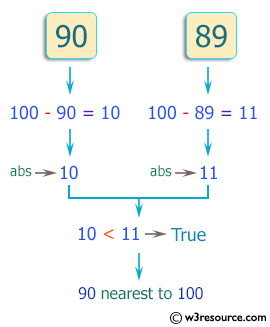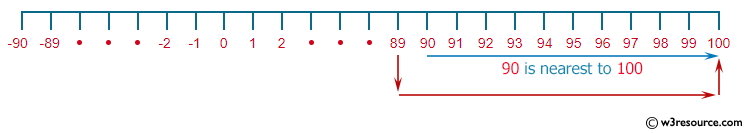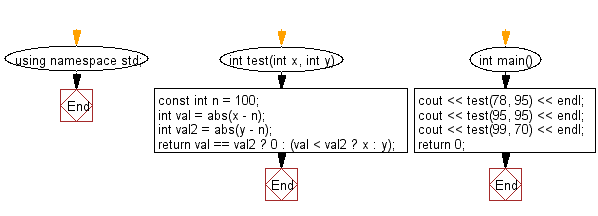﻿ C++ : Check if number nearest to the value 100 among two# C++ Exercises: Check which number nearest to the value 100 among two given integers

## C++ Basic Algorithm: Exercise-19 with Solution

Write a C++ program to check which number nearest to the value 100 among two given integers. Return 0 if the two numbers are equal.

Sample Solution:

C++ Code :

``````#include <iostream>
using namespace std;

int test(int x, int y)
{
const int n = 100;
int val = abs(x - n);
int val2 = abs(y - n);

return val == val2 ? 0 : (val < val2 ? x : y);
}

int main()
{
cout << test(78, 95) << endl;
cout << test(95, 95) << endl;
cout << test(99, 70) << endl;
return 0;
}
``````

Sample Output:

```95
0
99
```

Pictorial Presentation:Flowchart:C++ Code Editor: